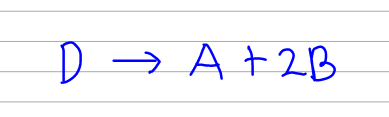# Problem: For A + 2B → 2C  Kc= 2.79                    For 2C → D  Kc= 0.106Calculate the value of the equilibrium constant for the reaction:D → A + 2BKc=?

###### FREE Expert Solution
100% (208 ratings)
###### FREE Expert Solution

So our first goal here is to set up the first 2 given reactions so that when we add them up, it will result in the reaction below.So we look at them 1 at a time.

100% (208 ratings)###### Problem Details

For A + 2B → 2C  Kc= 2.79

For 2C → D  Kc= 0.106

Calculate the value of the equilibrium constant for the reaction:

D → A + 2B

Kc=?

What scientific concept do you need to know in order to solve this problem?

Our tutors have indicated that to solve this problem you will need to apply the Chemical Equilibrium concept. You can view video lessons to learn Chemical Equilibrium. Or if you need more Chemical Equilibrium practice, you can also practice Chemical Equilibrium practice problems.

What is the difficulty of this problem?

Our tutors rated the difficulty ofFor A + 2B → 2C  Kc= 2.79                    For 2C → D  Kc=...as medium difficulty.

How long does this problem take to solve?

Our expert Chemistry tutor, Dasha took 3 minutes and 45 seconds to solve this problem. You can follow their steps in the video explanation above.

What professor is this problem relevant for?

Based on our data, we think this problem is relevant for Professor Betancourt's class at TXSTATE.